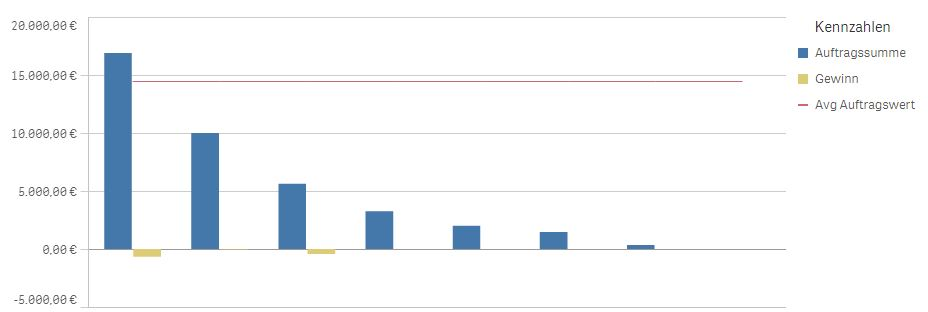# New to Qlik Sense

Discussion board where members can get started with Qlik Sense.

Highlighted
Contributor

## Average value of orders

Hi all together,

I try to achieve a diagram, that looks like this.I already got the diagramm, but what is missing is the average value. My dimension is "Projektleiter" and my measure is "Auftragswert", which is the value of a "Projekt". I tried to achieve a structure like seen above with the following formula:

((sum(Auftragswert))/Count(Projekt))*Count(distinct Projektleiter)

My graph looks like this:Is there any easy way to get the average amount as seen above?

Thanks for the help.

Regards,

Tom Müller

Tags (2)
1 Solution

Accepted SolutionsMVP & Luminary

## Re: Average value of orders

You could try it with:

((sum(total Auftragswert))/Count(total Projekt))*Count(distinct total Projektleiter)

- Marcus

4 RepliesMVP & Luminary

## Re: Average value of orders

You could try it with:

((sum(total Auftragswert))/Count(total Projekt))*Count(distinct total Projektleiter)

- Marcus

Contributor

## Re: Average value of orders

Thanks for the reply, now my graph looks like expected, but the value of the AVG function is too high.Do you have an idea, what could be the reason? I am wondering myself whether it is necessary to incloude the Count (total Projekt), may there be another possibilty? I believe the mistakes is caused by this part.

Honored Contributor II

## Re: Average value of orders

hi,

would be able to share the mock, so that we can check. tks

Contributor

## Re: Average value of orders

Thank you, I got it by myself. ((sum(total Auftragswert))/Count(distinct total Projektleiter) works.